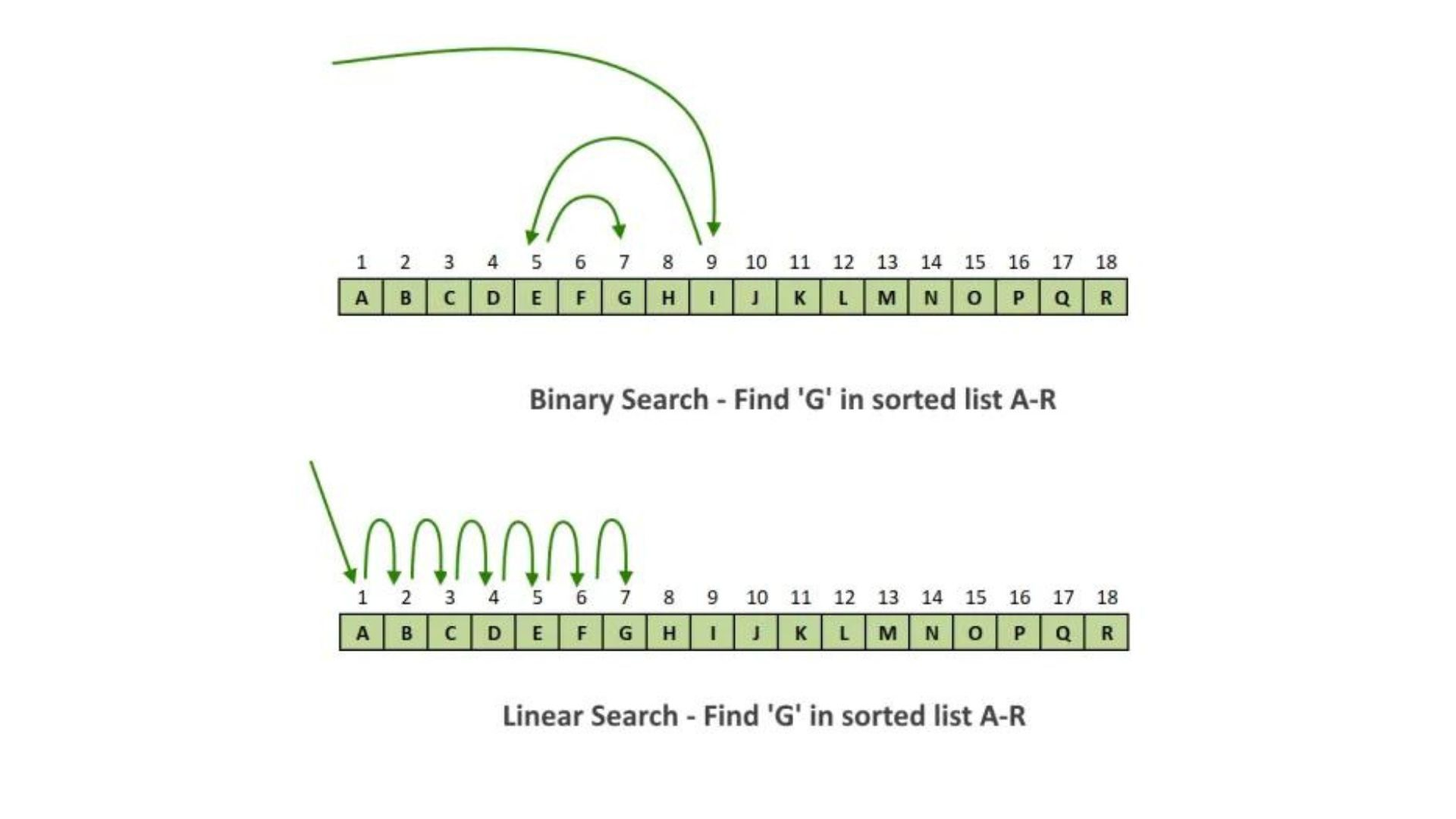# Searching Algorithm and its Types## Introduction

As software professionals, we must conduct computer searches for information much as we do in daily life. We cannot afford to spend a lot of time searching for information, thus information retrieval should be completed quickly. Therefore, we want some effective search methods or algorithms that can quickly find a specific piece of information and present it to the user so that they may go on to other activities. When looking for information, we mostly use two basic searching approaches. These consist of:

1. Search Linear
2. Binary Search

We will thoroughly examine both of these search strategies in this article.

The easiest to use and most fundamental search method is this one. In a linear search, each component of the data set is compared linearly with the key to be searched. On linear data structures, this method is effective.

Let us consider the following array:

• The seven-element array is shown above.
• The key value will indeed be evaluated for each element starting with element 0 if we wish to search for key = 23.
• The precise location will also be returned once the key element and the member in the array match.
• As the key value in this instance fits the value at position, 4, it will be returned.

Code

 #include #include using namespace std;int main(){   int myarray = {21,43,23,54,75,13,5,8,25,10};     int key,loc;    cout<<"The input array is"<>key;    for (int i = 0; i< 10; i++)     {         if(myarray[i] == key)          {             loc = i+1;            break;         }          else          loc = 0;     }      if(loc != 0)     {         cout<<"Key found at position "<

Binary search is a method for looking for a key that employs the "split and conquer" strategy. It employs a linear list of items that are sorted. In terms of accuracy and processing time, binary search is more effective. The linear search strategy is rarely utilized since it is slower and more laborious. A linear search is slower than a binary search. The essential prerequisite for a binary search function is the sorted list. The list is continually split in half in the binary search technique, and the key element is sought on both sides of the list until the key is discovered.

For Example, let us take the following sorted array of 10 elements.

• Let's imagine the array has to be searched for the key = 21.
• Let's figure out where the array's centre is -> Mid = 0+9/2 = 4
• Key = 21
• We'll first make a comparison between the key value and the [mid] element. The element number at mid is discovered to be 21.
• Our conclusion is that key = [mid]. So, the key is discovered.
• key = 25
• We start by contrasting the main value with the mid. Therefore, (21 25), we will immediately look for the key in the upper part of the array. For the top half of the array, we shall once more determine the midpoint.
• Mid = 4+9/2 = 6
• The value is 25 at position [mid].

The key element and the mid element are now being compared. We have located the key at position [mid] because (25 == 25). We repeatedly split the array, and we choose which half to look for the key in by matching the key element with the middle.

Code

 #include #include using namespace std;int binarySearch(int myarray[], int beg, int end, int key)  {      int mid;      if(end >= beg) {             mid = (beg + end)/2;          if(myarray[mid] == key)          {              return mid+1;          }          else if(myarray[mid] < key) {              return binarySearch(myarray,mid+1,end,key);          }          else {              return binarySearch(myarray,beg,mid-1,key);          }      }      return -1;   }   int main ()  {      int myarray = {5,8,10,13,21,23,25,43,54,75};      int key, location=-1;       cout<<"The input array is"<>key;      location = binarySearch(myarray, 0, 9, key);      if(location != -1)  {          cout<<"Key found at location "<
write your code here: Coding Playground

## Conclusion

The searching methods enable us to look for information that has been saved on a computer so that the user may go on to other information processing chores. Although the linear search strategy is straightforward and easier, it is not often employed. Since the binary search approach is quicker and more effective, it is frequently used.